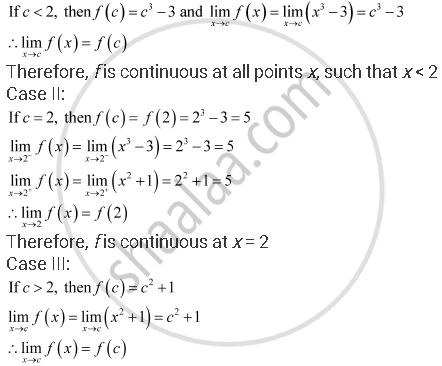Share

# Find All Points of Discontinuity Of F, Where F Is Defined by F(X) = {(X^3 - 3, If X < = 2),(Xsqrt2 + 1, If X > 2) - CBSE (Commerce) Class 12 - Mathematics

#### Question

Find all points of discontinuity of f, where f is defined by f(x) = {(x^3 - 3, if x <= 2),(x^2 + 1, if x > 2):}

#### Solution

The given function f is  f(x) = {(x^3 - 3, if x < = 2),(x^2 + 1, if x > 2):}

The given function f is defined at all the points of the real line.

Let c be a point on the real line.

Case I:Therefore, f is continuous at all points x, such that x > 2

Thus, the given function f is continuous at every point on the real line.

Hence, has no point of discontinuity.

Is there an error in this question or solution?

#### APPEARS IN

NCERT Solution for Mathematics Textbook for Class 12 (2018 to Current)
Chapter 5: Continuity and Differentiability
Q: 11 | Page no. 159

#### Video TutorialsVIEW ALL 

Solution Find All Points of Discontinuity Of F, Where F Is Defined by F(X) = {(X^3 - 3, If X < = 2),(Xsqrt2 + 1, If X > 2) Concept: Concept of Continuity.
S